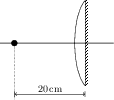# Combinations of Mirrors and Thin Lenses

## Problems from IIT JEE

Problem (IIT JEE 2006):A point object is placed at a distance of 20 cm from a thin plano-convex lens of focal length 15 cm. The plane surface of the lens is now silvered. The image created by the system is at,

1. 60 cm to the left of the system
2. 60 cm to the right of the system
3. 12 cm to the left of the system
4. 12 cm to the right of the system

Solution: Substitute $u={-20}\;\mathrm{cm}$ and $f={15}\;\mathrm{cm}$ in the lens formula, \begin{align} \label{tua:eqn:1} {1}/{v}-{1}/{u}={1}/{f}, \end{align} to get the image distance $v={60}\;\mathrm{cm}$. The image $I_1$ by the lens is formed 60 cm to the right of the system. Before converging, the rays are reflected by the plane mirror i.e., this image act as an object for the plane mirror. The image $I_2$ by the plane mirror is formed 60 cm towards the left of the system.The reflected rays, while going back, are refracted again by the lens i.e., image by the mirror acts as an object for the lens. This time, rays are travelling from the right to the left and hence the first focus of the lens is relevant. Thus, $u={-60}\;\mathrm{cm}$ and $f={-15}\;\mathrm{cm}$. Substitute these values in first equation to get $v={-12}\;\mathrm{cm}$ i.e., the image $I_3$ is formed 12 cm to the left of the system.